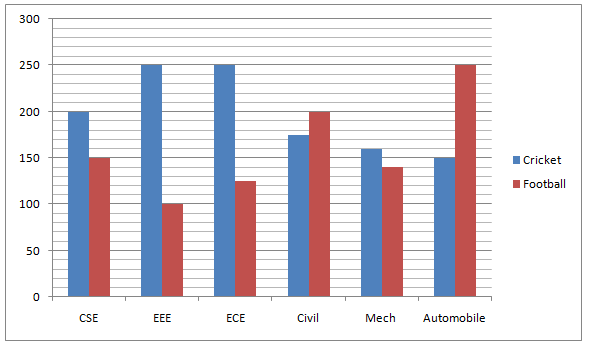# SBI Clerk Prelims 2021 Quantitative Aptitude Questions (Day-09)

Dear Aspirants, Our IBPS Guide team is providing new series of Quantitative Aptitude Questions for SBI Clerk Prelims 2020 so the aspirants can practice it on a daily basis. These questions are framed by our skilled experts after understanding your needs thoroughly. Aspirants can practice these new series questions daily to familiarize with the exact exam pattern and make your preparation effective.

Start Quiz

Mixture and allegation

1) Some quantity of rice costing Rs.30/kg is mixed with some quantity of rice costing Rs.35/kg. If final mixture costs Rs.32/kg, then find the ratio of quantity in which they are mixed?

A.2: 5

B.3: 2

C.1: 6

D.6: 5

E.None of these

Number system

2) On dividing a number by 42, we get 10 as remainder. On dividing the same number by 6, what will be the remainder?

A.4

B.2

C.6

D.8

E.None of these

Ratio and proportion

3) Rs.270 contained in a box consists of Rs.1, Rs.50 paise and Rs.25 paise coins in the ratio 2: 3: 4. What is the number of 25 paise coins?

A.120

B.180

C.240

D.260

E.None of these

Permutation and combination

4) In how many ways can the letters of the word ‘PLANTS’ be arranged when A, N are always together?

A.120

B.240

C.180

D.190

E.None of these

Time, speed and distance

5) The speed of car A and car B is 126 Km/hr and 90 Km/hr respectively. The time required by car B is 2 hours more than that of car A. Find total distance.

A.630 Km

B.540 Km

C.810 Km

D.270 Km

E.None of these

Bar graph

Directions (6-10): Study the following information carefully and answer the questions given below.

The given bar graph shows the number of boys who like two different games in six different departments from SRM College.6) What is the total number of boys who like both cricket and Football from CSE and EEE together?

A.700

B.600

C.800

D.750

E.None of these

7) What is the difference between the total number of boys like Cricket and Football together from Civil and the number of boys who like both games from Automobile department?

A.20

B.25

C.30

D.35

E.None of these

8) What is the total number of boys who likes Football from all the departments together?

A.935

B.945

C.955

D.965

E.None of these

9) Total number of boys who like Cricket and Football from Mech department is what percent of the total number of boys who like Cricket and Football from ECE department?

A.60%

B.70%

C.80%

D.75%

E.65%

10) What is the average number of boys who like Cricket from all the departments together except civil department?

A.202

B.210

C.212

D.227

E.None of these

x/y = (35 – 32)/(32 – 30) = 3/2

Let us take the number be x and quotient be y,

x = 42y + 10

= (6 * 7)y + 6 + 4

= 6 (7y + 1) + 4

Therefore, the number divided by 6 we get 4 as reminder.

(or)

Remainder on dividing by 42 = 10

Remainder on dividing the same number by 6 i.e 10/6 = 4 = Remainder

Ratio of the values of coins = 2: 3/2: 4/4 = 4: 3: 2

Sum = 4 + 3 + 2 = 9

Value of 25 paise coins = 2/9 * 270 = Rs.60

Number of 25 paise coins = 60 * 4 = Rs.240

PLANTS has 6 letters.

Number of ways A, N can be arranged 2! ways

Taking AN as 1 letter, then there are 5 letters.

Required nmber of ways = 5! * 2! = 240

Ratio of Speeds, A: B = 126: 90 = 7: 5

Ratio of time, A: B = 5: 7

Difference between ratio of time = 7 – 5 = 2 (which is equal to given diff.)

Therefore, Distance = 126 × 5 = 90 × 7 = 630 Km

Required total = 200 + 150 + 250 + 100 = 700

Total number of boys like both games from Civil = 175 + 200 = 375

Total number of boys like both games from Automobile = 150 + 250 = 400

Difference = 400 – 375 = 25

Required total = 150 + 100 + 125 + 200 + 140 + 250 = 965

Required percentage = (160 + 140)/(250 + 125) * 100

= 80%